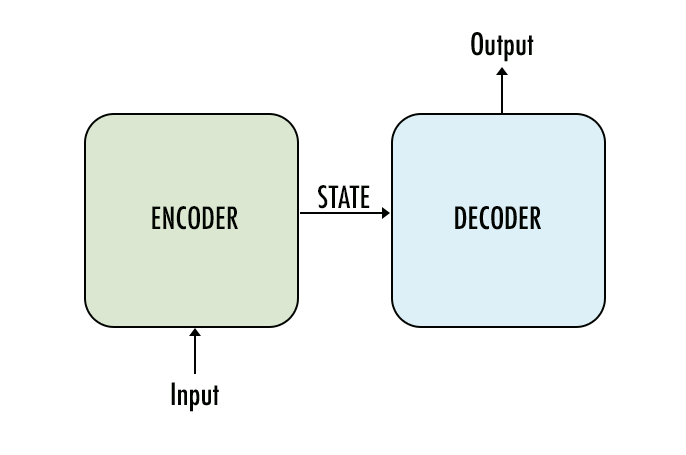# txt2txt

An extremely easy to use and very configurable seq2seq implementation with Attention for text to text use cases.

Working with seq2seq tasks in NLP, I realised there aren’t any easy to use, simple to understand and good performing libraries available for this. Though libraries like FairSeq or transformer are available they are in general either too complex for a newbie to understand or most probably overkill (and are very tough to train) for simple projects.

This module provides pre-built seq2seq model with Attention that performs excellently on most of the “simple” NLP taks. (Tested with Punctuation correction, transliteration and spell correction).

# Examples# Installation

``````# Install tensorflow or tensorflow-gpu separately
pip install txt2txt
``````

# Usage

``````# Training a model
from txt2txt import build_params, build_model, convert_training_data

input_data = ['123', '213', '312', '321', '132', '231']
output_data = ['123', '123', '123', '123', '123', '123']

build_params(input_data = input_data, output_data = output_data, params_path = 'params', max_lenghts=(10, 10))

model, params = build_model(params_path='params')

input_data, output_data = convert_training_data(input_data, output_data, params)

checkpoint = ModelCheckpoint('checkpoint', monitor='val_acc', verbose=1, save_best_only=True, mode='max')
callbacks_list = [checkpoint]

model.fit(input_data, output_data, validation_data=(input_data, output_data), batch_size=2, epochs=20, callbacks=callbacks_list)

# Inference (Beam)
from txt2txt import build_model, infer
model, params = build_model(params_path='params')